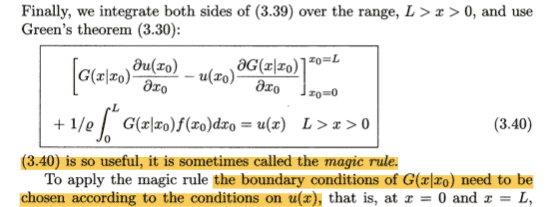# Green's function for the wave equation

• I
Gold Member
Hi, I'm reading "Wave Physics" by S. Nettel and in chapter 3 he introduces the Green's function for the 1-dimensional wave equation. Using the separation of variables method he restricts his attention to the spatial component only. Let ##u(x)## be the spatial solution to the wave equation and ##G(x|x_0)## the Green's function. The author now states the following:

Let us now suppose that it is ##u(x)## that is specified at an end point. Then we must choose ##G(x|x_o)## to vanish at that end point.

I don't really understand this connection. Can someone help me ?

Thanks
Ric

Staff Emeritus
Homework Helper
Gold Member
You are looking to write down the solution on the form
$$u(x) = \int G(x|x_0) \kappa(x_0) dx_0 + f(x)$$
where ##\kappa## is the inhomogeneity in your wave equation and ##f(x)## solves the homogeneous wave equation and satisfies your boundary conditions. If ##G(x|x_0)## does not vanish at the boundary, then the integral will generally not be zero there and your function will not satisfy the boundary conditions.

•Cryo
Gold Member
In the book I'm reading there is a slightly different version of the solution:(forget about the ##\frac 1 {\rho}##)

BTW I also do not understand your answer. If, for example, ##k## vanishes at the end point the integral could also be zero and the boundary condition would be verified.

Staff Emeritus
•